# TI-30XS MultiView™ Scientific Calculator

Powerful, four-line scientific calculator for high school math and science exploration.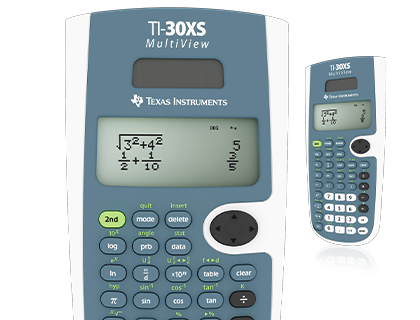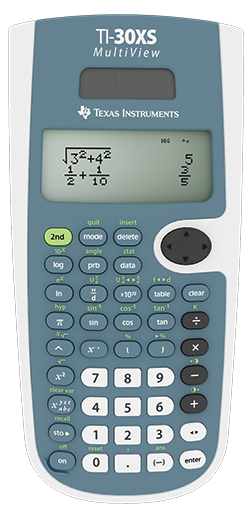#### Key features

• Four-line display
• One- and two-variable statistics
• MathPrint™ feature
• Fraction/decimal conversion
• Fraction math
• Edit, cut and paste entries
• Solar and battery powered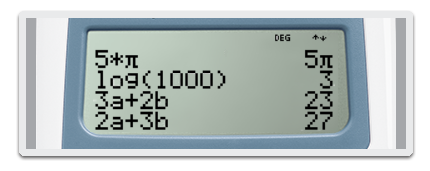## View more calculations at a time

Four-line display allows you to enter more than one calculation, compare results and explore patterns, all on the same screen.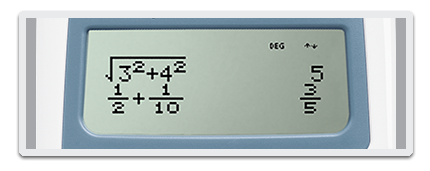## MathPrint™ feature

Use the MathPrint™ feature to display expressions, symbols, and fractions just as they appear in textbooks.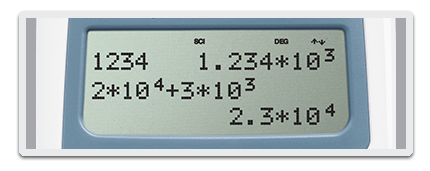## Scientific notation output

View scientific notation with the proper superscripted exponents and see the output in scientific notation.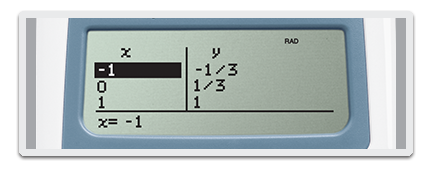## Explore (x,y) table of values

Students can easily explore an (x,y) table of values for a given function, automatically or by entering specific x values.## TI-30XS MultiView™ Scientific Calculator Specifications#### Built-in functionality

• Review and edit previous entries via a scrollable home screen
• Paste inputs or outputs into new calculations
• MathPrint™ entry and output mode for viewing calculations in math notation, including answers in terms of π, square roots and fraction
• CLASSIC mode for similar entry and compatibility with previous two-line scientifics
• Symbolic notation of π
• Toggle key to change the form of answers between exact and decimal approximation
• Stacked fractions and fraction functions
• Fraction/decimal/percent conversions
• Change between improper fractions and mixed numbers
• Automatic simplification of fractions
• Random number and random integer generator
• Central MODE menu for selecting calculator mode settings
• Functions accessed directly through keys or through pull-down menus
• Negation key
• One constant operator feature
• Combinations and permutations
• Trigonometry
• Hyperbolics
• Logs and antilogs
• %, x², ¹/x, yˆx, π, x!
• Fixed decimal capability
• (x,y) table feature with Auto and Ask-x options
• Basic Data/List Editor with three lists
• List formulas
• One- and two-variable statistics with permanent stat variable input storage
• EOS: (Equation Operating System)
• Up to eight pending operations
• Up to 23 levels of parentheses
• Error recovery capability
• Auto Power Off
• Quick/easy reset of calculator via 2 key press or menu for exam purposes
• Seven memory variables. (x,y,z,t,a,b,c)
• Scientific and engineering notation
• x10n key for quick input of numbers in powers of 10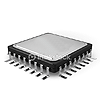#### Hardware

• Four-line × 16-character, easy-to-read LCD display
• Dual power (solar and battery)
• Hard plastic, color-coded keys
• Non-skid rubber feet
• Snap-on protective hard case
• Teacher Kit Available (includes 10 calculators, storage caddy, Teacher's Guide in English and Spanish, calculator poster and transparency)
• Quick-reference card#### Support## TI-30XS MultiView™ Scientific Calculator Accessories

Extend the power of your calculator with accessories for the TI-30XS MultiView™ scientific calculator.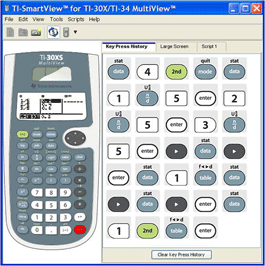## TI-SmartView™ Emulator Software

This simple software complements the TI-30X/TI-34 MultiView™ scientific calculators, letting the teacher project a representation of the calculator’s display to the entire class. It is an ideal demonstration tool for leading classroom instruction of maths and science concepts.